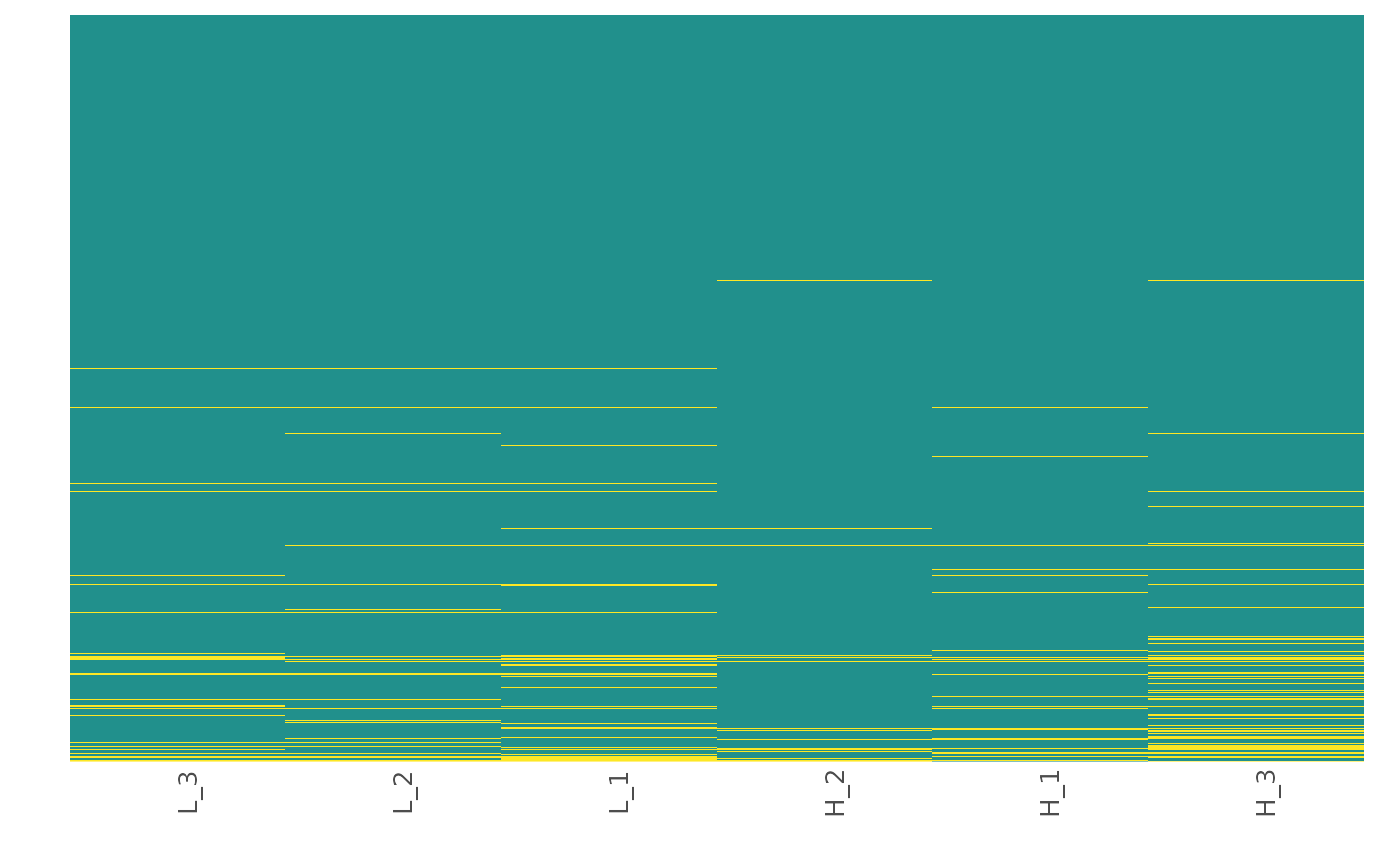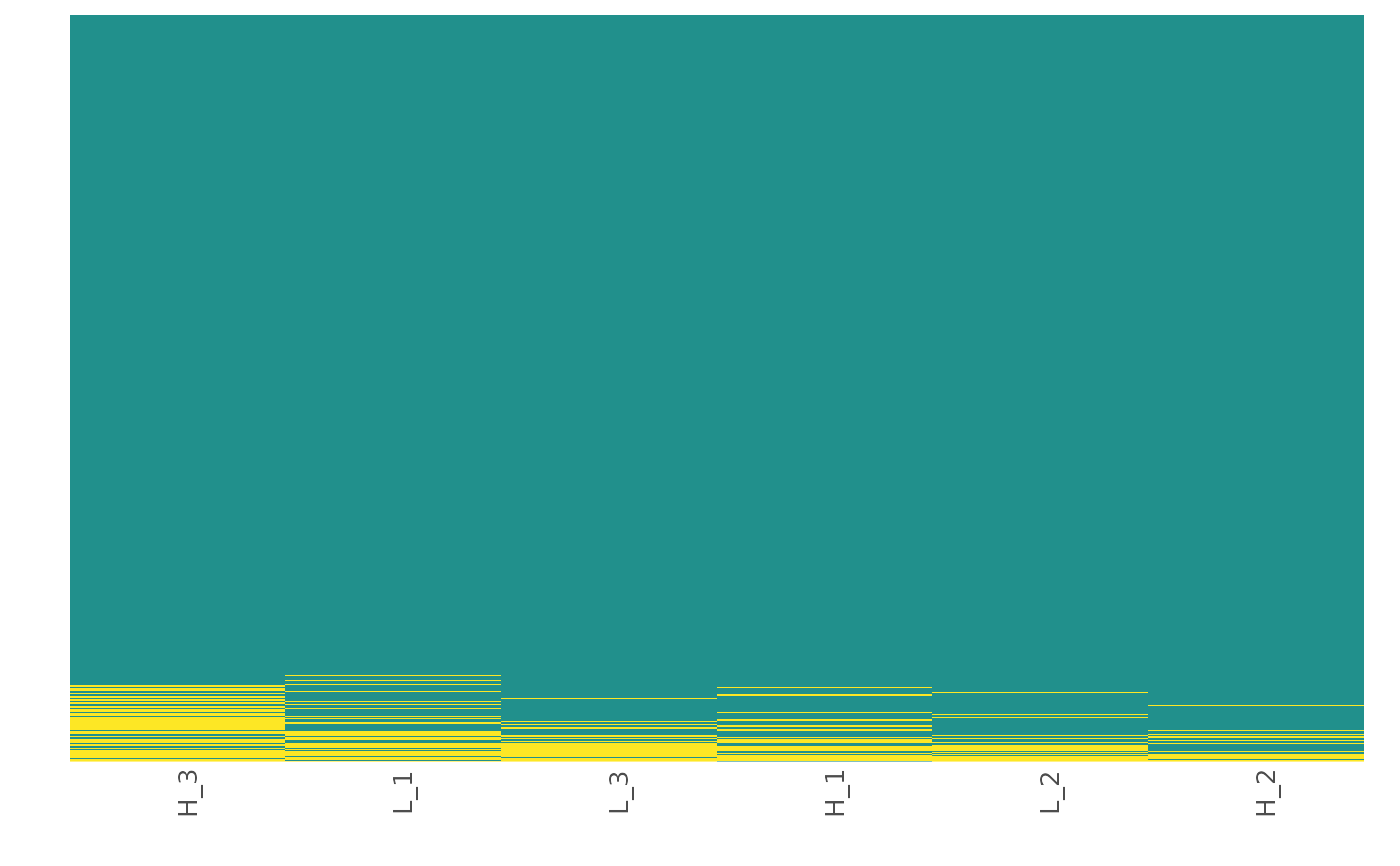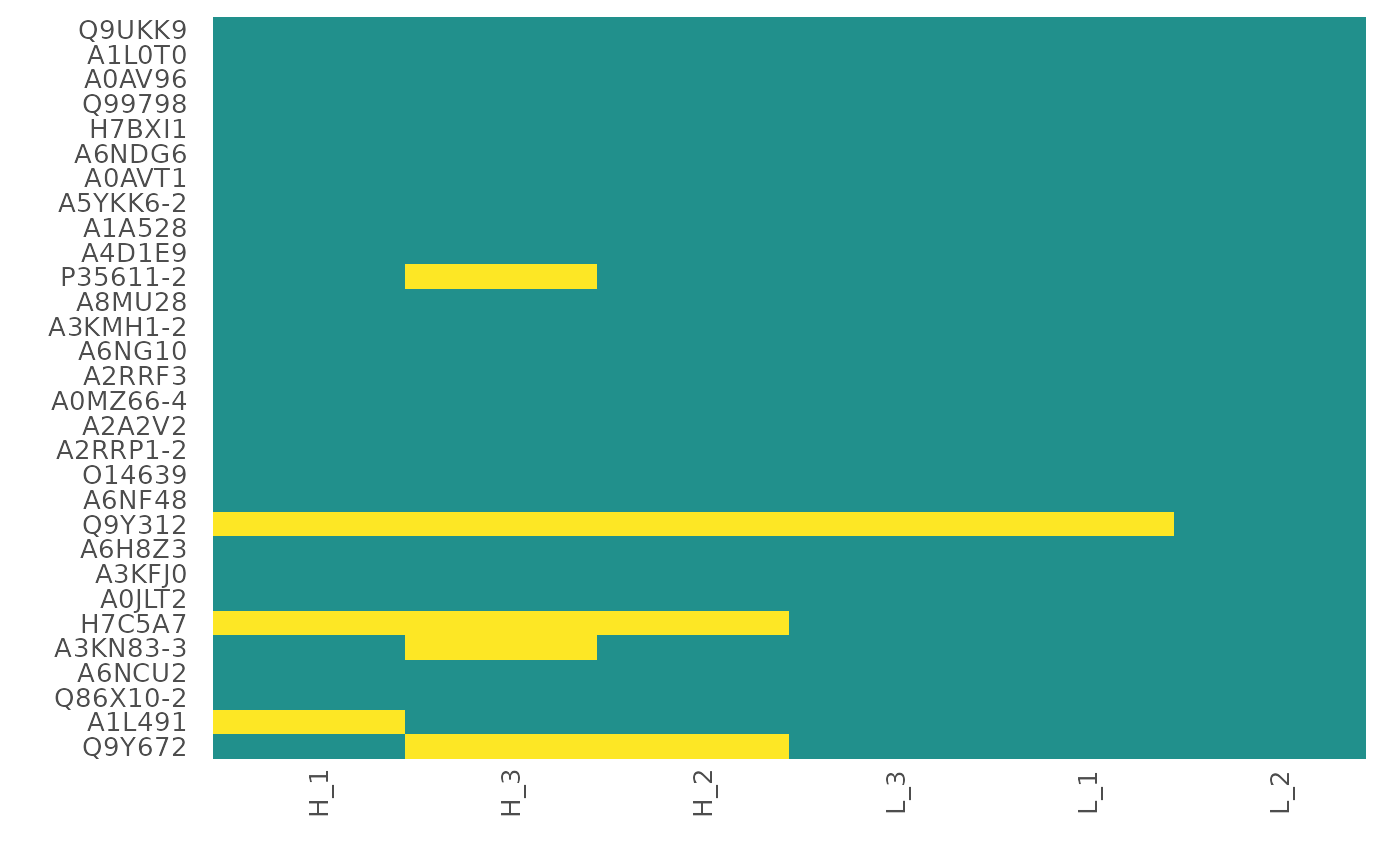This function visualizes the patterns of missing value occurrence using a heatmap.

## Usage

heatmap_na(
raw_df,
protein_range,
sample_range,
reorder_x = FALSE,
reorder_y = FALSE,
x_fun = mean,
y_fun = mean,
palette = "viridis",
label_proteins = FALSE,
text_size = 10,
save = FALSE,
file_type = "pdf",
file_path = NULL,
file_name = "Missing_data_heatmap",
plot_width = 15,
plot_height = 15,
dpi = 80
)

## Arguments

raw_df

A raw_df object (output from create_df).

protein_range

The range or subset of proteins (rows) to plot. If not provided, all the proteins (rows) in the data frame will be used.

sample_range

The range of samples to plot. If not provided, all the samples (columns) in the data frame will be used.

reorder_x

Logical. If TRUE samples on the x axis are reordered using the function given in x_fun. Default is FALSE.

reorder_y

Logical. If TRUE proteins in the y axis are reordered using the function given in y_fun. Default is FALSE.

x_fun

Function to reorder samples along the x axis. Possible options are mean and sum. Default is mean.

y_fun

Function to reorder proteins along the y axis. Possible options are mean and sum. Default is mean.

palette

Viridis color palette option for plots. Default is "viridis". See viridis for available options.

label_proteins

If TRUE proteins on the y axis will be labeled with their Majority Protein IDs. Default is FALSE.

text_size

Text size for axis labels. Default is 10.

save

Logical. If TRUE saves a copy of the plot in the directory provided in file_path.

file_type

File type to save the heatmap. Default is "pdf".

file_path

A string containing the directory path to save the file.

file_name

File name to save the heatmap. Default is "Missing_data_heatmap".

plot_width

Width of the plot. Default is 15.

plot_height

Height of the plot. Default is 15.

dpi

Plot resolution. Default is 80.

## Value

A ggplot2 plot object.

## Details

This function visualizes patterns of missing value occurrence using a heatmap. The user can choose to reorder the axes using the available functions (x_fun, y_fun) to better understand the underlying cause of missing data.

create_df

## Author

Chathurani Ranathunge

## Examples

## Generate a raw_df object with default settings. No technical replicates.
raw_df <- create_df(
prot_groups = "https://raw.githubusercontent.com/caranathunge/promor_example_data/main/pg1.txt",
exp_design = "https://raw.githubusercontent.com/caranathunge/promor_example_data/main/ed1.txt"
)
#> 0 empty row(s) removed.
#> 0 empty column(s) removed.
#> 80 protein(s) (rows) only identified by site removed.
#> 65 reverse protein(s) (rows) removed.
#> 42 protein potential contaminant(s) (rows) removed.
#> 1923 protein(s) identified by 2 or fewer unique peptides removed.
#> Zeros have been replaced with NAs.
#> Data have been log-transformed.

## Missing data heatmap with default settings.
heatmap_na(raw_df)## Missing data heatmap with x and y axes reordered by the mean (default) of
## protein intensity.
heatmap_na(raw_df,
reorder_x = TRUE, reorder_y = TRUE
)## Missing data heatmap with x and y axes reordered by the sum of
## protein intensity.
heatmap_na(raw_df,
reorder_x = TRUE, reorder_y = TRUE, x_fun = sum,
y_fun = sum
)## Missing data heatmap for a subset of the proteins with x and y axes
## reordered by the mean (default) of protein intensity and the y axis
## labeled with protein IDs.
heatmap_na(raw_df,
protein_range = 1:30,
reorder_x = TRUE, reorder_y = TRUE,
label_proteins = TRUE
)